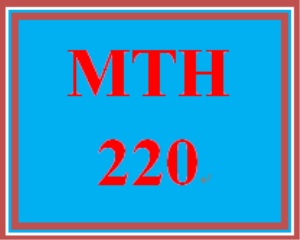# MTH 220 Week 4 Signature Assignment: Sequence

PLDZ-4432 In Stock
\$ 5.00 USD
Description

MTH 220 Week 4 Signature Assignment: Sequence

https://uopcourses.com/category/mth-220/

MTH 220 Week 4 Signature Assignment: Sequence

Open the Microsoft® Excel® file you created in Week 3 with the best fit line.

Select “Save As” to create a new version of this workbook.

Delete your commentary from Week 3 and add new commentary per below:

How does the graph of a line compare to an arithmetic sequence? What does the slope represent? How would you find the first term?

Use your best fit equation to make a formula for the nth term of an arithmetic sequence. The year will represent the number of the term, and your data value will be the value at that term.

Use your arithmetic equation to predict what the 2,050th term will be. Do you think this is a good estimate of what the real value will be? Why or why not?

Challenge Option

Open your Microsoft® Excel® file from Week 2 with your exponential graph.

Click “Save As” to create a new version of this file.

Delete the old commentary.

How does the graph of an exponential equation compare to a geometric sequence? How would you find the common ratio? How would you find the first term (assuming the year is the number of the term)?

Use your best fit equation to make a formula for the nth term of a geometric sequence. The year will represent the number of the term, and your data value will be the value at that term.

Use your geometric equation to predict what the 2,050th term will be. Do you think this is a good estimate of what the real value will be? Why or why not?

Save your Microsoft® Excel® file, including your old graphed equation and new commentary. Make sure you do not delete your files, as you will need them in subsequent weeks. Your assignment will be graded with the Week 4 Grading Guide.

Click the Assignment Files tab to submit your assignment.

Recent Reviews Write a Review
0 0 0 0 reviews